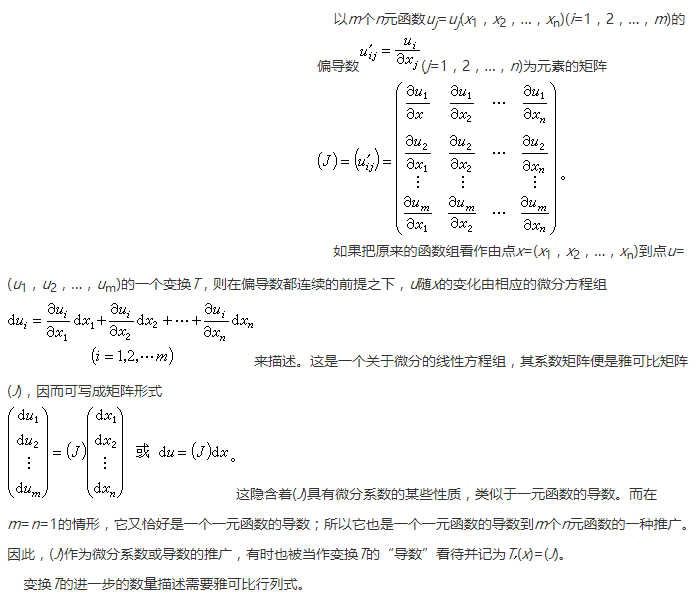0%

# I. 雅可比矩阵

$\begin{bmatrix} \frac{\partial y_1}{\partial x_1} \cdots \frac{\partial y_1}{\partial x_n} \\ \vdots \ddots \vdots \\ \frac{\partial y_m}{\partial x_1} \cdots \frac{\partial y_m}{\partial x_n} \end{bmatrix}$

$F({\bf{x} }) \approx F({\bf{p} }) + {J_F}({\bf{p} }) \cdot ({\bf{x} } \cdot {\bf{p} })$